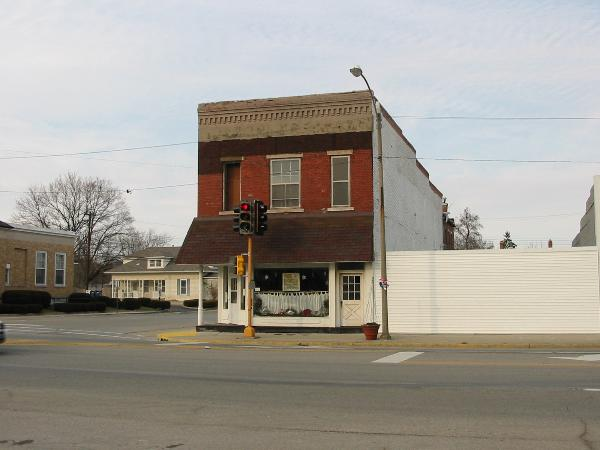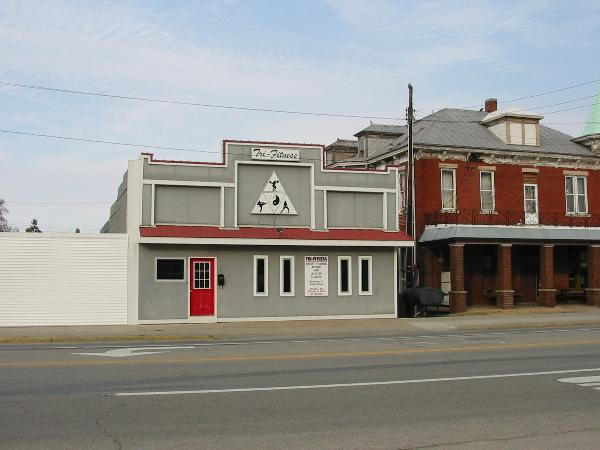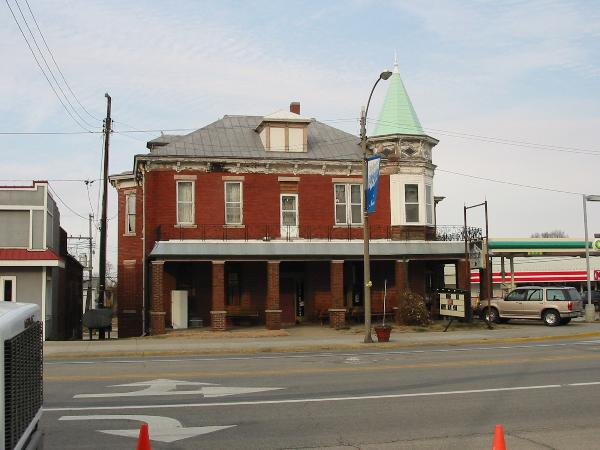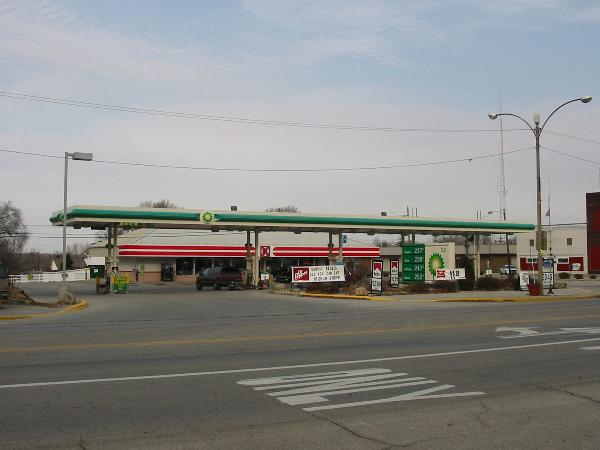North Side Square Newton Illinois 2006
North Side Square Newton Illinois 2006
North Side Square Newton Illinois 2006
North Side Square Newton Illinois 2006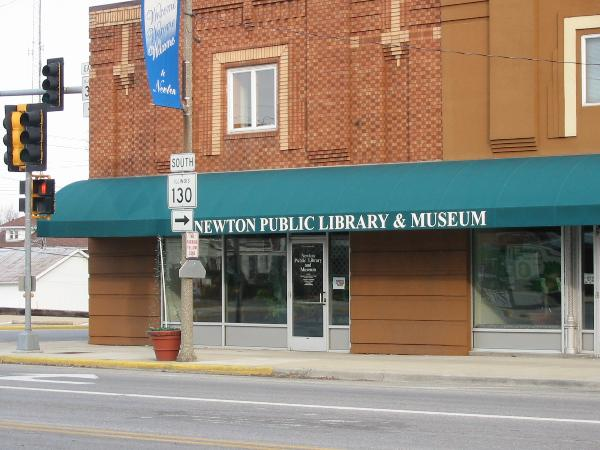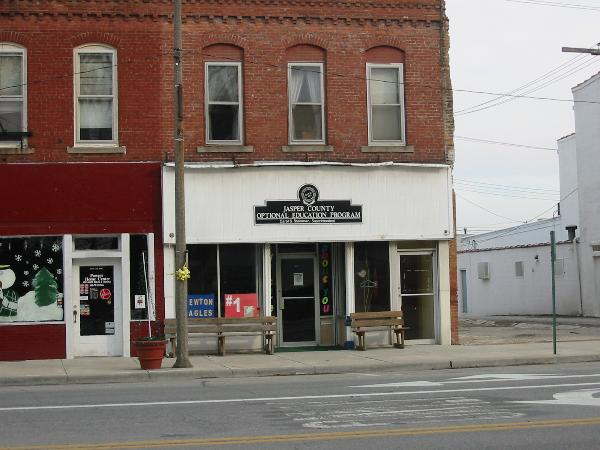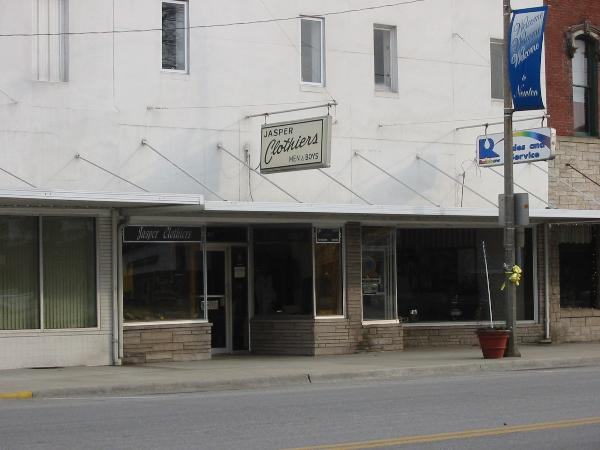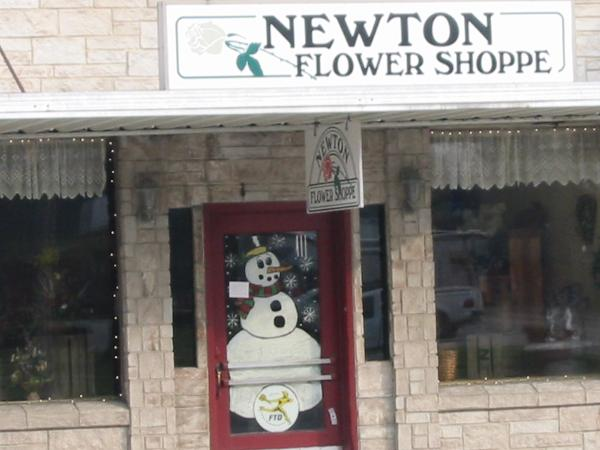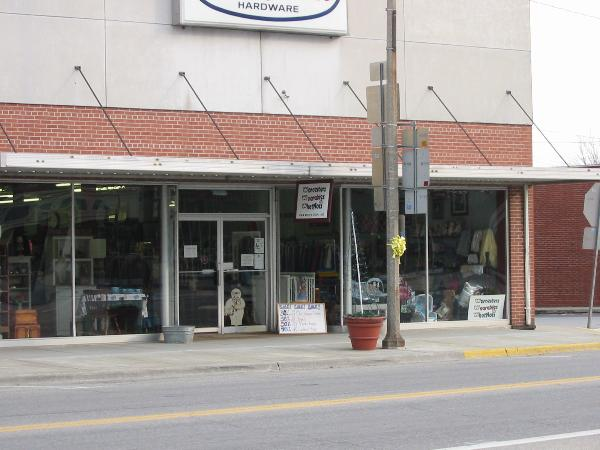East Side Square Newton Illinois 2006
East Side Square Newton Illinois 2006
East Side Square Newton Illinois 2006
East Side Square Newton Illinois 2006
East Side Square Newton Illinois 2006
East Side Square Newton Illinois 2006
East Side Square Newton Illinois 2006
East Side Square Newton Illinois 2006
East Side Square Newton Illinois 2006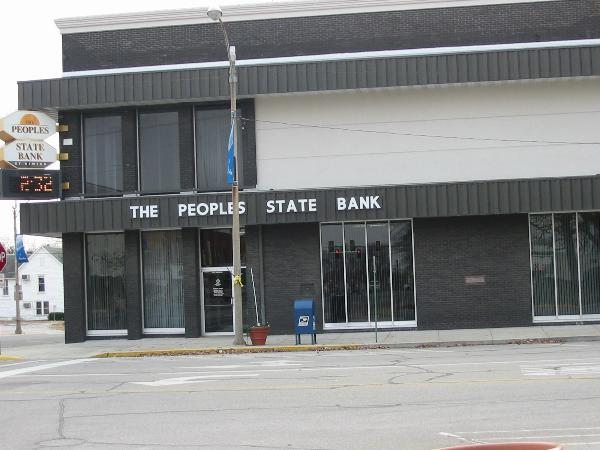South Side Of Square Newton Il 2006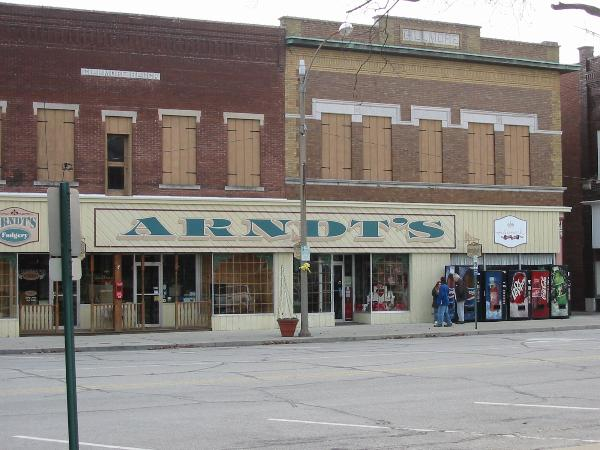South Side Of Square Newton Il 2006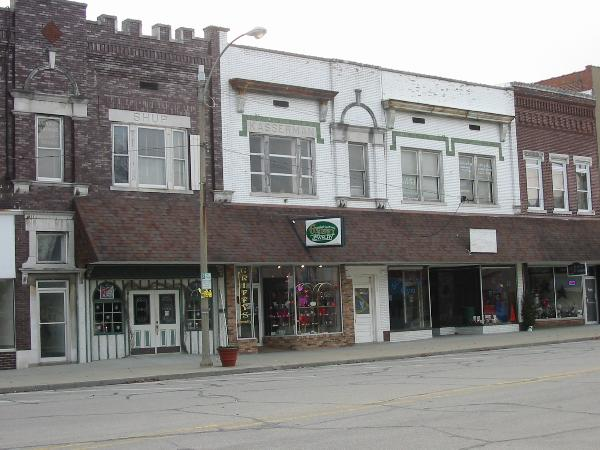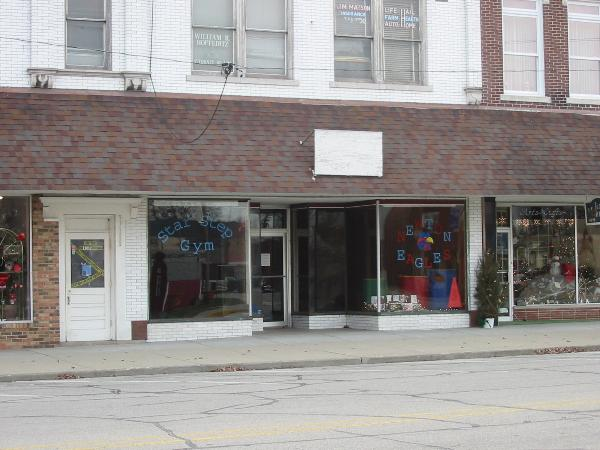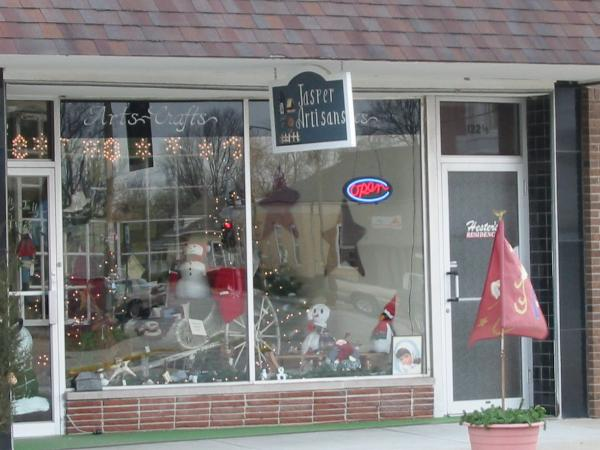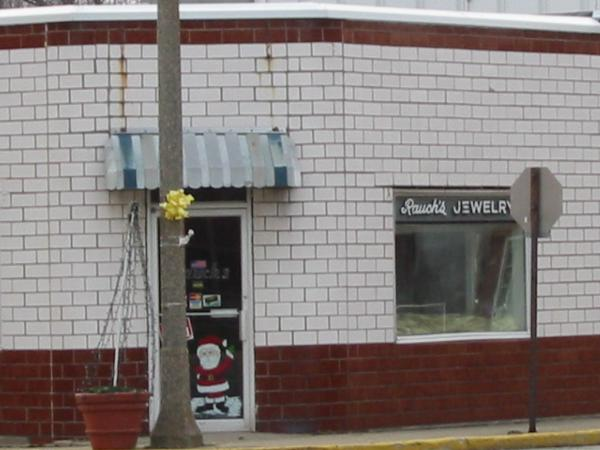West Side of Square Newton Il 2006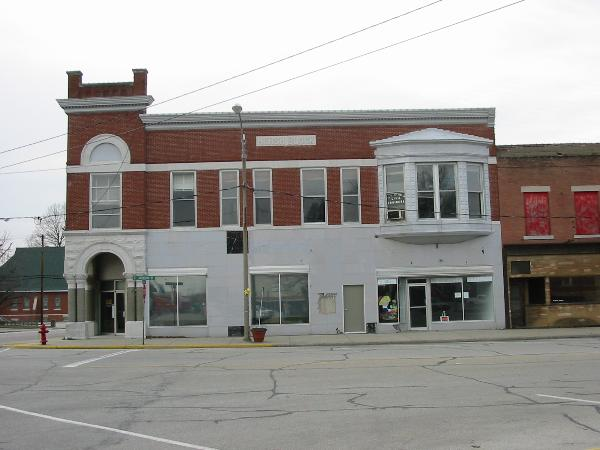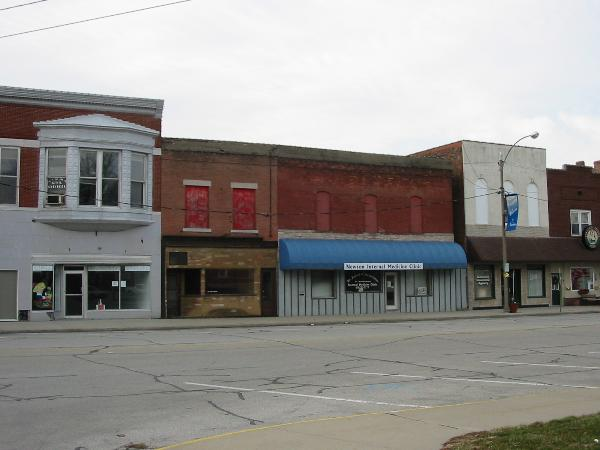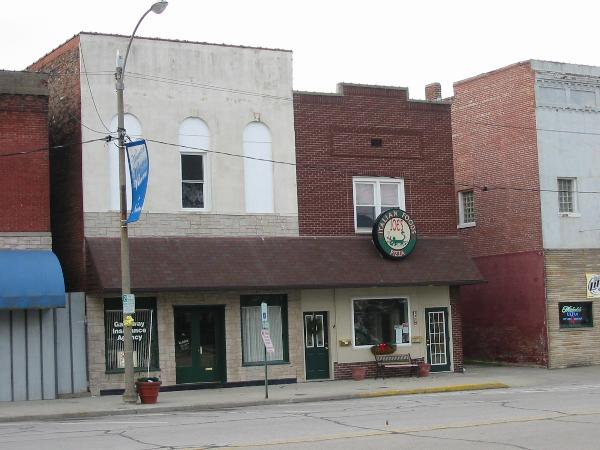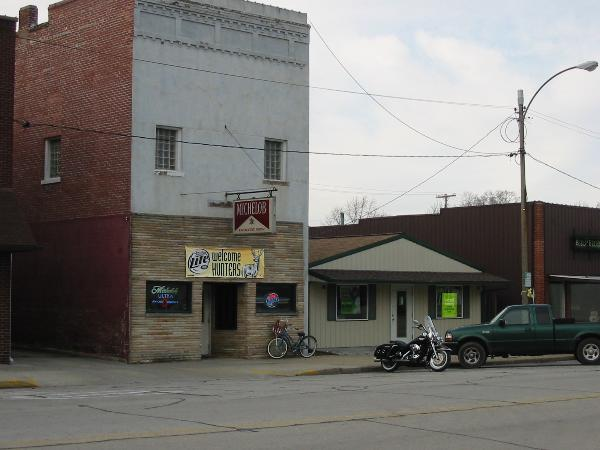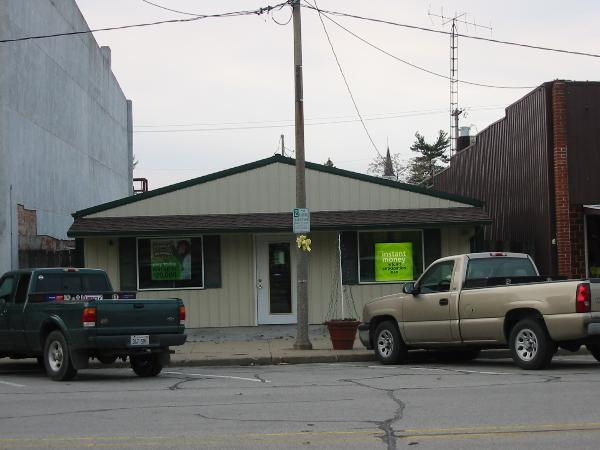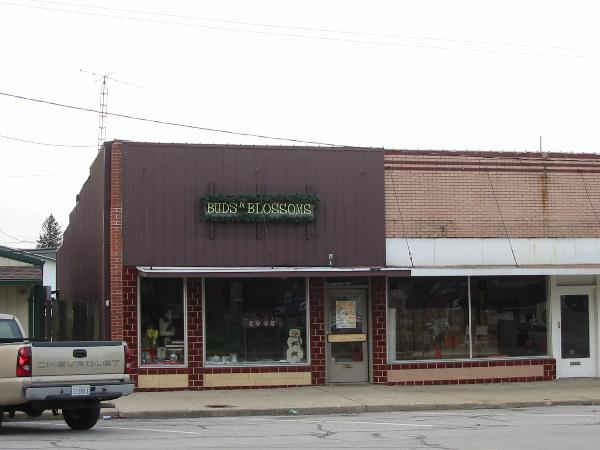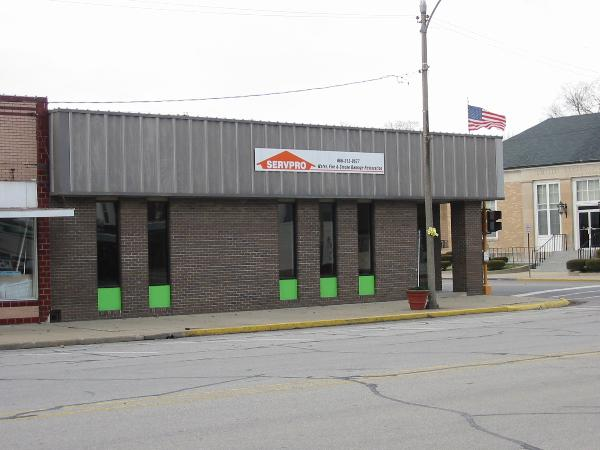These Pictures were taken around the Newton Illinois square in 2006